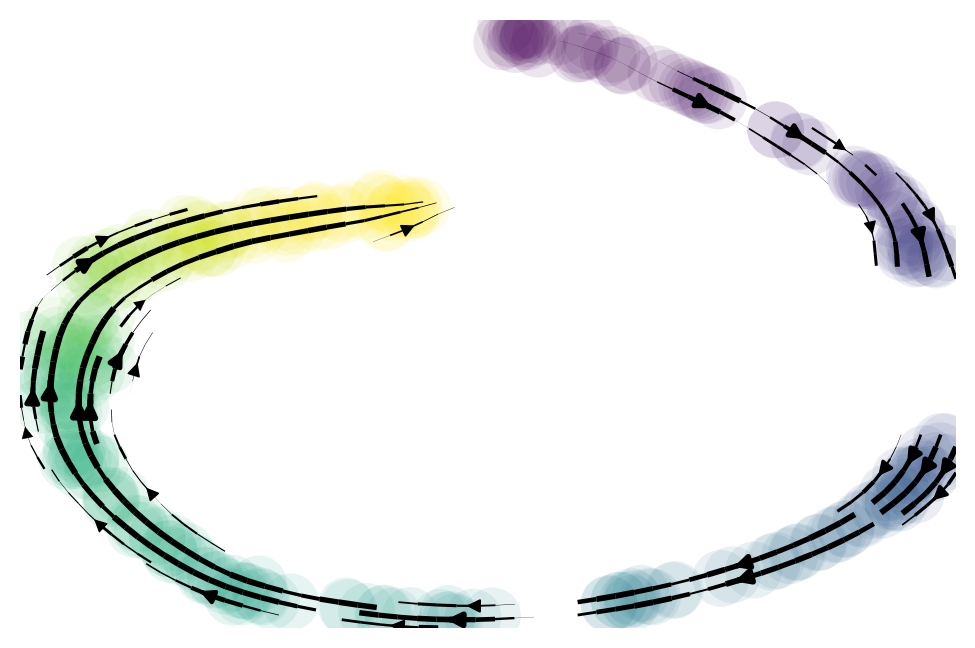# scvelo.datasets.simulation¶

`scvelo.datasets.``simulation`(n_obs=300, n_vars=None, alpha=None, beta=None, gamma=None, alpha_=None, t_max=None, noise_model='normal', noise_level=1, switches=None, random_seed=0)

Simulation of mRNA splicing kinetics.

Simulated mRNA metabolism with transcription, splicing and degradation. The parameters for each reaction are randomly sampled from a log-normal distribution and time events follow the Poisson law. The total time spent in a transcriptional state is varied between two and ten hours.Returns